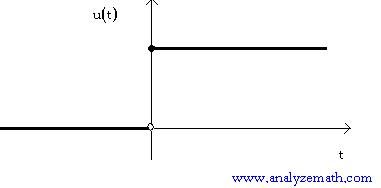# Table of Fourier Transforms

## Definition of Fourier Transforms

If f(t) is a function of the real variable t, then the Fourier transform F(ω) of f is given by the integral
F(ω) = -∞+∞ e - j ω t f(t) dt

where j = √(-1), the imaginary unit.
In what follows, u(t) is the unit step function defined by
u(t) = 1 for t ≥ 0 and u(t) = 0 for t < 0. (see figure below).## Table of Fourier Transforms

 f(t) F(ω) u(t) e -a t , a > 0 1 / (a + j ω) 1 for - a ≤ t ≤ a and 0 otherwise 2 sin (ω a) / ω A (constant) 2 π A δ (ω) δ (t) 1 δ (t - a) e - j ω a cos (a t) π [ δ(ω + a) + δ(ω - a) ] sin (a t) - j π [ δ (ω - a) - δ (ω + a) ] e j a t 2 π [δ(ω - a) ] f '(t) j ω F (ω) f ''(t) (j ω) 2 F(ω) t f(t) j d [F(ω)] / dω t 2 f(t) j 2 d 2[F(ω)] / dω 2